# Unit of Electrical Energy

The unit of electric power is watt, and hence unit of electrical energy is watt – second since energy is the product of power and time. The watt – seconds is referred as joules. One joule means the work required to be done for transferring one-ampere current in one second from one point to another point of potential difference one volt. So one-joule electrical energy equals to the product of one volt, one ampere and one second.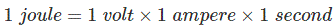Joule which equals to one watt – second is a very small unit of energy, and it is very difficult to measure a practically consumed electrical energy by this unit.

## Commercial Unit of Electrical Energy

To resolve this problem of measuring practically consumed electrical energy, a commercial unit of electrical energy comes into the picture. The commercial unit of electrical energy is a bigger unit of electrical energy. This is watt-hour.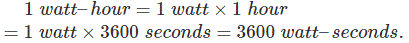Another bigger unit of electrical energy is kilowatt-hours or kWh. This equals to 1000 X one watt-hour.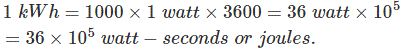### Relation between Unit of Electrical Energy and Unit of Mechanical Energy

The basic definition of a unit of mechanical energy is the amount of work to be done to move a body one meter with a force of one newton. This unit of mechanical energy is joule. Again one joule of electrical energy equals to one watt – second. Now, we can write,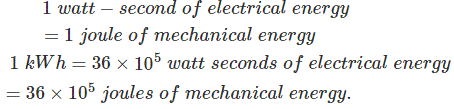### Relation between Unit of Electrical Energy and Unit of Heat Energy

Heat is another form of energy which is wildly used in engineering. The unit of heat energy is calorie, British thermal unit and centigrade heat unit. One calorie of heat energy is the amount of heat required to raise the temperature of one gram water to one degree centigrade.

Practically calorie is a very small unit of heat that is why we often use kilocalorie instead. One kilocalorie is the amount of heat required to raise the temperature of 1 kg of water to 1oC.

British thermal unit is the amount of heat required to raise the temperature of 1 pound of water to 1oF.

Centigrade heat unit is the amount of heat required to raise the temperature of 1 pound of water to 1oC.

The mechanical work to be done for raising the temperature of one gram of water to one degree centigrade is 4.18 joules. We can say one calorie is equivalent to 4.18 joules.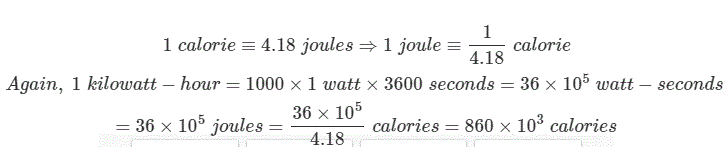#### Electrical Energy Equivalent of Centigrade Heat Unit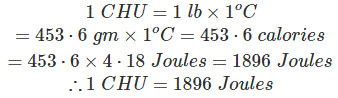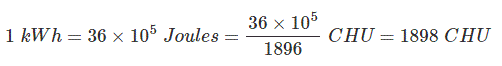#### Electrical Energy Equivalent of British Thermal Unit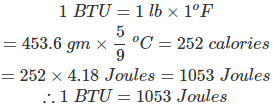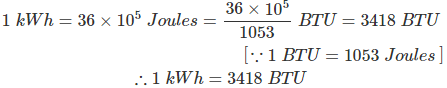Want To Learn Faster? 🎓
Get electrical articles delivered to your inbox every week.
No credit card required—it’s 100% free.